Levels »

# Wrong Way!

### The Catacombs, Elevation: -2.4m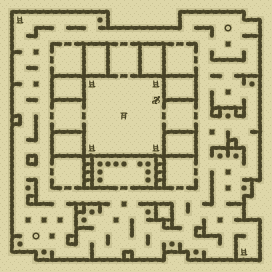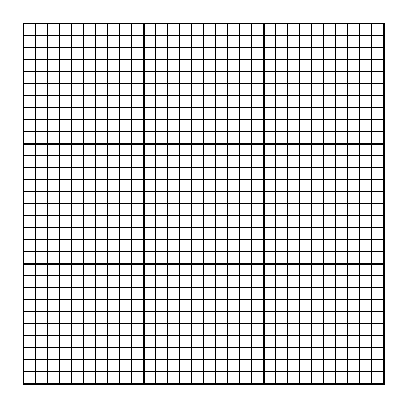### Items

•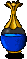Clear Blue Potion (6, 12)
•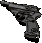Walther P4 Pistol (18, 11) (on Behrens)
•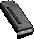19 Walther P4 Magazines (149 rounds) (30, 2) x8 - (8, 7) x8 - (13, 7) x8 - (20, 7) x8 - (20, 7) x8 - (28, 9) x8 - (17, 11) x8 - (18, 11) x8 (in Walther P4 Pistol) (on Behrens) - (18, 11) x8 (on Behrens) - (18, 11) x8 (on Behrens) - (18, 11) x8 (on Behrens) - (18, 11) x8 (on Behrens) - (18, 11) x8 (on Behrens) - (16, 15) x5 - (6, 19) x8 - (20, 19) x8 - (6, 27) x8 - (6, 27) x8 - (11, 30) x8
•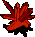Orange Crystal (14, 20) (50 uses left)

•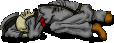(18, 11) Behrens
•Walther P4 Pistol
•Walther P4 Magazine x8
•Walther P4 Magazine x8
•Walther P4 Magazine x8
•Walther P4 Magazine x8
•Walther P4 Magazine x8
•Walther P4 Magazine x8

### Monsters

•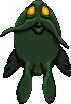19 Nightmares (6, 5) - (8, 5) - (22, 5) - (13, 6) - (15, 6) - (8, 7) - (20, 7) - (7, 12) - (22, 12) - (7, 14) - (22, 14) - (8, 19) - (22, 19) - (16, 20) - (6, 21) - (12, 21) - (14, 21) - (16, 21) - (22, 21)
•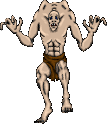17 Ghouls (Random rate: 1) (8, 1) - (24, 1) - (2, 2) - (2, 9) - (11, 9) - (17, 9) - (10, 10) - (18, 10) - (2, 15) - (10, 16) - (18, 16) - (11, 17) - (17, 17) - (12, 20) - (26, 22) - (22, 26) - (16, 27)
•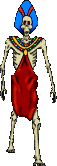10 Zombies (Random rate: 3) (25, 1) - (30, 5) - (26, 11) - (28, 19) - (12, 20) - (7, 26) - (1, 27) - (4, 28) - (11, 28) - (16, 30)

### Locations

• 29 Silver key-operated door openers (7, 3) - (14, 3) - (21, 3) - (7, 5) - (14, 5) - (21, 5) - (4, 6) - (6, 6) - (22, 6) - (24, 6) - (14, 7) - (14, 9) - (4, 13) - (6, 13) - (8, 13) - (10, 13) - (18, 13) - (20, 13) - (22, 13) - (24, 13) - (4, 20) - (6, 20) - (22, 20) - (24, 20) - (7, 21) - (21, 21) - (7, 23) - (14, 23) - (21, 23)
• 2 Save runes(27, 2) - (3, 28)